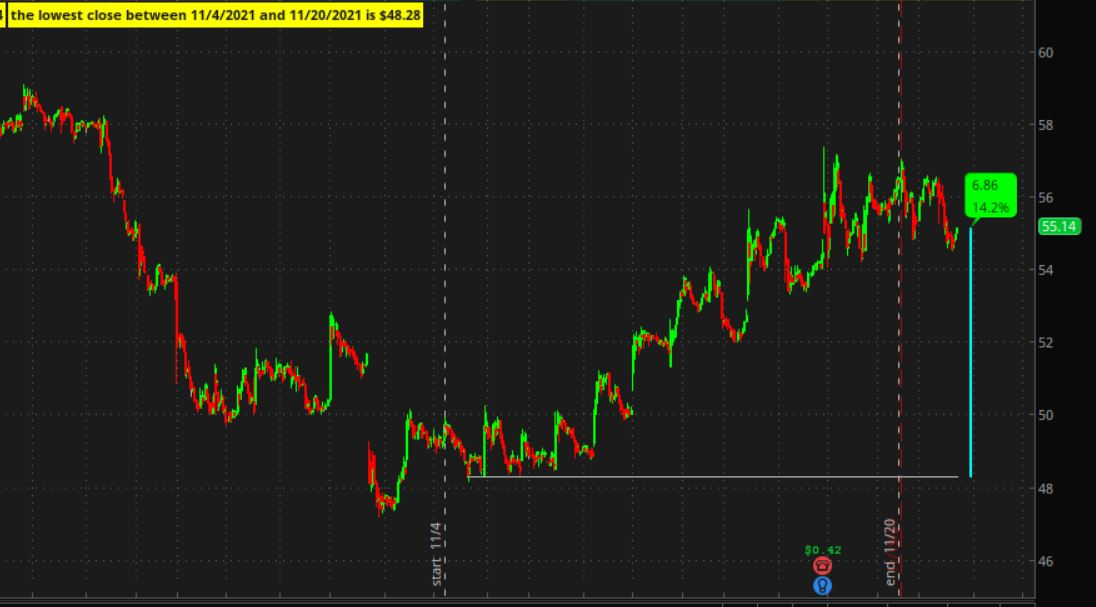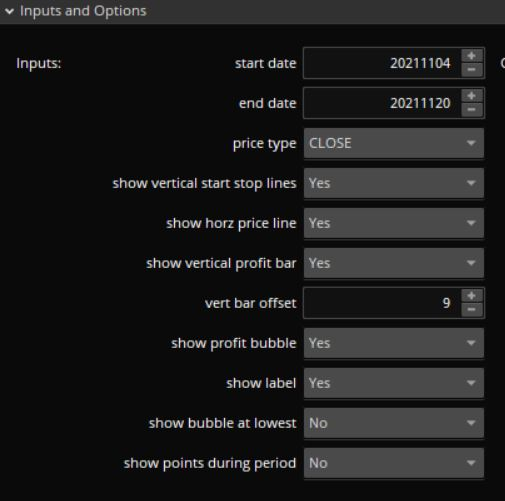# Find the lowest close price between two points

#### jassimraja

##### New member
I would like to have a ThinkScript that will find the lowest close price between two points in ThinkorSwim and then compare that value with the current close. If the current close is lower than the lowest close between two points then display it on a scanner.

Solution
Thanks @halcyonguy, is there a way to find the lowest close between two dates for example; the lowest close between July 1st 2021 to September 30th 2021? And then compare that value with the current bar close?
Find a price level between 2 dates and compare it to the current close

This will,
..find the lowest close between 2 dates, user inputs. (not the low)
..a yellow label displays the 2 dates and the low price.
..draw a horizontal line from the low point, to the last bar.
..draw a colored bar a few spaces past the last bar, from the low close and current close. calling it a profit bar.
....its placement is determined by this offset vert_bar_offset = 9;
....i changed the color of this bar to cyan or yellow. i didn't...
I would like to have a ThinkScript that will find the lowest close price between two points in ThinkorSwim and then compare that value with the current close. If the current close is lower than the lowest close between two points then display it on a scanner.

this can guide you on 1 way to do it.
this draws horizontal lines from peaks and valleys. use the variables that hold the price levels for those lines, by comparing them to the current bar close.

just in case the post moves, here is the link

this can guide you on 1 way to do it.
this draws horizontal lines from peaks and valleys. use the variables that hold the price levels for those lines, by comparing them to the current bar close.

just in case the post moves, here is the link
Thanks @halcyonguy, is there a way to find the lowest close between two dates for example; the lowest close between July 1st 2021 to September 30th 2021? And then compare that value with the current bar close?

Thanks @halcyonguy, is there a way to find the lowest close between two dates for example; the lowest close between July 1st 2021 to September 30th 2021? And then compare that value with the current bar close?
Find a price level between 2 dates and compare it to the current close

This will,
..find the lowest close between 2 dates, user inputs. (not the low)
..a yellow label displays the 2 dates and the low price.
..draw a horizontal line from the low point, to the last bar.
..draw a colored bar a few spaces past the last bar, from the low close and current close. calling it a profit bar.
....its placement is determined by this offset vert_bar_offset = 9;
....i changed the color of this bar to cyan or yellow. i didn't want a user mistaking it for a real candle.
..a bubble near the profit bar will display the price difference of current close and previous lowest close, and the % difference.
....the bubble color changes, green or red, and the orientation, up or down.

If the start date is less than the dates on the chart, a light blue label appears up with a warning message.
( i prefer to use bright colored labels for user warnings, instead of assert() )

If the expansion area is set to 0, you won't see the bubble or profit bar.

As i tested and debugged, i added a couple of things....
This started out simple, but i like to have visual things happen on the chart, so i added the bar after the last bar.

note the formulas to extract date info.

I started from this post from @SleepyZ. It might give you some other ideas.

Code:
``````# between2dates_01
# halcyonguy   21-23-21

def bn = barnumber();
def na = double.nan;
input start_date = 20211104;
input end_date = 20211120;

def date1 = if bn == 1 then getYYYYMMDD() else date1;

def chart1year = Round(date1/10000, 0);
def chart1month = Round((date1 % 10000) / 100, 0);
def chart1day = (date1 % 100);

addlabel(start_bad , " xxx  start date is before the first date on chart , " + (chart1month + "/" + chart1day + "/" + AsPrice(chart1year)) + "  xxx" , color.cyan);
#addchartbubble(1, low*0.996, "s " + start_date + "\n1 " + date1 + "\n" + (start_date < date1) + "\n" + start_bad, color.cyan, no);

def yearstart = Round(start_date/10000, 0);
def monthstart = Round((start_date % 10000) / 100, 0);
def daystart = (start_date % 100);
#addLabel(1, "start date: " + monthstart + "/" + daystart + "/" + AsPrice(yearstart), color.white);

def yearend = Round(end_date/10000, 0);
def monthend = Round((end_date % 10000) / 100, 0);
def dayend = (end_date % 100);
#addLabel(1, "end date: " + monthend + "/" + dayend + "/" + AsPrice(yearend), color.white);

input price_type = close;

def isbetween = if Between( GetYYYYMMDD(), start_date, end_date) then 1 else 0;

def between_first = ( !isbetween and isbetween );
def between_last = ( isbetween and !isbetween[-1] );

input show_vertical_start_stop_lines = yes;
addverticalline(show_vertical_start_stop_lines and between_first, "start  " +  monthstart + "/" + daystart, color.light_gray);
addverticalline(show_vertical_start_stop_lines and between_last, "end  " + monthend + "/" + dayend, color.light_gray);

# if it is the 1st bar in the isbetween period, set it to the close, don't compare to the previous bar.
# if it is any other bar in the isbetween period, compare the close to tempmin.
def tempmin =
if between_first then price_type
else if isbetween then min( price_type , tempmin )
else na;

def mincls = lowestAll(if isbetween then tempmin else na);

# make a level to compare to current bar
def mincls2 = if bn == 1 then na
else if (isbetween and mincls == price_type) then mincls
else mincls2;

input show_horz_price_line = yes;
plot z1 = if (show_horz_price_line) then mincls2 else na;
#z1.SetPaintingStrategy(PaintingStrategy.POINTS);
z1.SetDefaultColor(Color.light_gray);
z1.setlineweight(1);
z1.hidebubble();

# ----------------------------------------------
# profit bar
# draw a bar, x bars after the last bar, to indicate the price change since the low of period
input show_vertical_profit_bar = yes;
input vert_bar_offset = 9;

# show_vertical_profit_bar and
def x1 = ( (!isnan(close[vert_bar_offset]) and isnan(close[(vert_bar_offset-1)]) ));
def profit = if x1 then round( close[vert_bar_offset] - mincls2[vert_bar_offset] , 2) else na;
def profit_per = round(100 * profit/mincls2[vert_bar_offset] , 1);
def profit_dir = sign( profit );

# +  green
# set open to lower level and close to higher level. but swap them in addchart() to draw a filled bar with grow color
def o1 = if (x1 and profit_dir == 1 and show_vertical_profit_bar) then mincls2[vert_bar_offset] else na;
def c1 = if (x1 and profit_dir == 1) then close[vert_bar_offset] else na;
def h1 = if x1 then c1 else na;
def l1 = if x1 then o1 else na;
# open / close rev to draw solid
# green up bar
#AddChart(growColor = Color.green, fallColor = Color.red, neutralColor = Color.gray, high = h1, low = l1, open = c1, close = o1, type = ChartType.CANDLE);
# cyan up bar
AddChart(growColor = Color.cyan, fallColor = Color.cyan, neutralColor = Color.gray, high = h1, low = l1, open = c1, close = o1, type = ChartType.CANDLE);

# -  red
def o2 = if (x1 and profit_dir == -1 and show_vertical_profit_bar) then mincls2[vert_bar_offset] else na;
def c2 = if (x1 and profit_dir == -1) then close[vert_bar_offset] else na;
def h2 = if x1 then c2 else na;
def l2 = if x1 then o2 else na;
# red down bar
#AddChart(growColor = Color.red, fallColor = Color.red, neutralColor = Color.gray, high = h2, low = l2, open = o2, close = c2, type = ChartType.CANDLE);
# yellow down bar
AddChart(growColor = Color.yellow, fallColor = Color.yellow, neutralColor = Color.gray, high = h2, low = l2, open = o2, close = c2, type = ChartType.CANDLE);

# +  green  yes ,  red no
input show_profit_bubble = yes;
addchartbubble( show_profit_bubble and x1, close[vert_bar_offset], profit + "\n" + profit_per + "%", (if profit_dir == 1 then color.green else color.red), (if profit_dir == 1 then 1 else 0) );

#-------------------------------------------------

input show_label = yes;
#addlabel(show_label, "the lowest close between " + start_date + " and " + end_date + " is \$" + mincls, color.yellow);
addlabel(show_label, "the lowest close between " + ( monthstart + "/" + daystart + "/" + AsPrice(yearstart) ) + " and " + (monthend + "/" + dayend + "/" + AsPrice(yearend)) + " is \$" + mincls, color.yellow);

# show a bubble at the lowest low
input show_bubble_at_lowest = no;
addchartbubble( (show_bubble_at_lowest and isbetween and mincls == price_type), low*0.996, mincls, color.yellow, no);

input show_points_during_period = no;
plot z2 = if (show_points_during_period and isbetween ) then ( high*1.01) else na;
z2.SetPaintingStrategy(PaintingStrategy.POINTS);
z2.SetDefaultColor(Color.light_gray);
z2.setlineweight(1);
z2.hidebubble();

# --------------------------------

# the dates used in  between()  are inclusive.  equal to or between the 2 dates.
# https://tlc.thinkorswim.com/center/reference/thinkScript/Functions/Others/Between
#``````

price moving up YY 15minsettingsLast edited:
•agirlhasnoname42 and jassimraja

### Not the exact question you're looking for?

87k+ Posts
177 Online## The Market Trading Game Changer

Join 2,500+ subscribers inside the useThinkScript VIP Membership Club
• Exclusive indicators
• Proven strategies & setups
• Private Discord community
• Exclusive members-only content
• 1 full year of unlimited support

What is useThinkScript?

useThinkScript is the #1 community of stock market investors using indicators and other tools to power their trading strategies. Traders of all skill levels use our forums to learn about scripting and indicators, help each other, and discover new ways to gain an edge in the markets.

How do I get started?

We get it. Our forum can be intimidating, if not overwhelming. With thousands of topics, tens of thousands of posts, our community has created an incredibly deep knowledge base for stock traders. No one can ever exhaust every resource provided on our site.

If you are new, or just looking for guidance, here are some helpful links to get you started.

What are the benefits of VIP Membership?